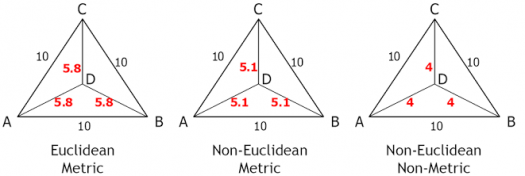# How Well Do You Know Euclidean Geometry?

10 Questions | Total Attempts: 49SettingsThe ancients attempted to understand the shape of the Earth and to use this understanding to solve problems in navigation over long distances. What have you learned of Euclidean Geometry?

• 1.
Who is the founder of the Euclid geometry?
• A.

Spanish mathematician; Euclid

• B.

Greek mathematician; Euclid..

• C.

Italian mathematician; Euclid

• D.

None of the above

• 2.
Euclid geometry is the plane and solid geometry commonly taught in secondary schools.
• A.

True

• B.

False

• C.

Indecisive

• D.

No Idea

• 3.
Euclidean geometry is an axiomatic system, in which all theorems are derived from a small number of axioms.
• A.

True

• B.

False

• C.

Indecisive

• D.

No Idea

• 4.
Hyperbolic geometry means the same thing as Euclidean geometry.
• A.

True

• B.

False

• C.

Indecisive

• D.

No Idea

• 5.
The three steps from solids to points are?
• A.

Solids – Surfaces – Lines – Points

• B.

Solids – Lines – Surfaces- Points

• C.

Lines – Surfaces – Solids – Points

• D.

None of the above.

• 6.
How many dimensions does a solid have?
• A.

1

• B.

2

• C.

3

• D.

4

• 7.
How many dimensions does a surface have?
• A.

1

• B.

2

• C.

3

• D.

4

• 8.
How many dimensions does a point have?
• A.

0

• B.

1

• C.

2

• D.

3

• 9.
What shape are side faces of a pyramid?
• A.

Triangle

• B.

Square

• C.

Polygons

• D.

Trapeziums

• 10.
The boundaries of solids are called?
• A.

Curves

• B.

Points

• C.

Surfaces

• D.

Lines

Related TopicsBack to top